## 3.2.2 Calling of module COLIB2

Preprocessor COLIXX  (see below as well as the simplified versions and module
 COLIBR) initiates a conversational call to the module for the case where the amount  of input data is little. In the general case, it seems simpler to call the module directly by writing a main program as follows:

• dimension the array M (in the blank common)
• declare the integer array NARET(5,NA) where NA is the number of coarse edges
• declare the integer array NTGRO(11,NBGRO) where NBGRO is the number of coarse elements
• declare the integer array NTRS(NBS) where NBS is the number of coarse vertices
• declare the integer array NTFR(6,NBFR) where NBFR is the number of coarse faces having a non-zero reference number (if NBFR = 0, NTFR is declared as NTFR(6,1))
• declare the real array XYZ(3,NBS) where NBS is the number of coarse vertices
• declare the real array XYZINT(3,LINT) where LINT is the total number of control points input by the user on the coarse edges (if LINT = 0, XYZINT is declared as XYZINT(3,1))
• call INITI
• call the module

CALL COLIB2 (M,NFNOPO,NINOPO,NDIMS,NBS,NA,NBGRO,
. NBFR,XYZ,NTRS,NARET,XYZINT,NTFR,NTGRO)

where

• M is the super array,
• NF(NI)NOPO is the support number of the O.D.S. NOPO and its level,
• NDIMS is the dimension of the output space, if NDIMS = 2, side Z is ignored (without control); this allows us to create segments, triangles or quadrilaterals in the R˛ space,
• NBS is the number of vertices in the coarse mesh,
• NA is the number of edges in the coarse mesh,
• NBGRO is the number of blocks (or elements) in the coarse mesh,
• NBFR is the number of faces to describe in order to assign a non-zero reference number to them,
• XYZ(3,.) is the coordinates of the coarse vertices (XYZ(J,I) is coordinate J of vertex I)
• NARET(5,.) is the array of coarse edges where
• NARET(1,I) is the number of the vertex which is the origin of edge I
• NARET(2,I) is the number of its end-point
• NARET(3,I) is the splitting code of the edge: 0 for a straight edge split automatically into segments of the same length; 1 if we input the intermediate points (see XYZINT)
• NARET(4,I) is the number of control points to create on the edge (end-points excluded)
• NARET(5,I) is the edge reference
• XYZINT(3,.) are the coordinates of the control points of the edges input by the user. For each edge I for which NARET(3,I) = 1, we enter these values as follows: XYZINT(J,I)is coordinate J of point I, where I is the global number of the point; we first count the points (end-points excluded) of the first edge thus described, followed by those of the second, etc.
• NTFR(6,.) is the array of the faces to specify where:
• NTFR(1,I) is the reference of face I
• NTFR(2,I) is its geometric code (3: triangle, 4: quadrilateral)
• NTFR(3,I) to NTFR(6,I) is the list of the vertices (0 for vertex '4' of a triangle)
• NTGRO(11,.) is the array of coarse elements where:
• NTGRO(1,I) is the geometric type of block I (2: segment, 3: triangle,
4: quadrilateral, 5: tetrahedron, 6: pentahedron, 7: hexahedron)
• NTGRO(2,I) to NTGRO(9,I) is the list of its vertices (0 for non-existing vertices)
• NTGRO(10,I) is the geometric type of the finite elements resulting from the splitting of the block (see remark below)
• NTGRO(11,I) is the sub-domain number of block I (therefore that of all the sub-elements created)

Remarks:

• The module checks the positiveness of the volumes of the elements created. In the case where one or several are negative, it signifies that either the edge control points are input badly (inversely, in particular), or the geometry of the block under consideration is too "distorted".
• In the present version, NTGRO(10,I) = NTGRO(1,I).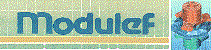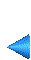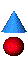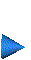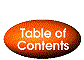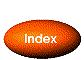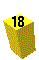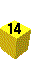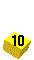Next: 3.2.3 Calling of the derived modules Up: 3.2 Multi-block method Prev: 3.2.1 Quick description of the method Index Contents